## ICSE Class 9 Physics Sample Question Paper 9 with Answers

Section -1
(Attempt all questions from this section)

Question 1.
(a) Name four fundamental quantities.
(b) Name the instrument which has the least count: (i) 0.1 mm, (ii) 0.01 mm.
(c) Two simple pendulums A and B have equal length, but their bobs weigh 150 gf and 200 gf respectively. What would be the ratio of their time periods ? Give reason for your answer.
(d) Two simple pendulums P and Q have length 4 m and 9 m respectively at a certain place. Which pendulum will have more oscillations in 1 minute ? Explain your answer.
(e) If time periods of two pendulums are in the ratio of 3 : 5, find the ratio of their lengths.
1. (a) Length, mass, time and temperature are examples of fundamental quantities.

(b) (i) Vernier callipers (ii) Screw gauge.

(c) The ratio of the time periods of pendulums A and B is 1 : 1. This is because time period does not depend on the weight of the bob.

(d) Since time period is directly proportional to the square root of effective length, $$(\mathrm{T} \propto \sqrt{l})$$
the time periods of P and Q are 2 sec $$(\sqrt{4})$$ and 3 sec $$(\sqrt{9})$$ respectively. Clearly P will complete 30 oscillations and Q will only complete 20 oscillations in 1 minute. Hence, pendulum P makes more oscillations.Question 2.
(a) A body starts from rest and moves for 5 seconds. It accelerates uniformly and its velocity changes to 25 m/s. Find the acceleration of the body.
(b) According to Newton’s third law, every force is accompanied by an equal and opposite force. How can a movement ever take place ?
(c) Why is Newton’s law of gravitation known as the universal law ?
(d) Select the scalars and vectors from the following : time, distance, acceleration, work, mass, force.
(e) “The value of ‘g’ remains same at all places on the earth’s surface.” Is this statement true ? Give reason for your answer.
(a) Given : u = 0 (body starts from rest), t = 5 s, v = 25 ms-1
$$a=\frac{v-u}{t}=\frac{25-0}{5}=5 \mathrm{~ms}^{-2}$$

(b) Action and reaction do not act on the same body, but act on different bodies and, hence motion is possible.

(c) Newton’s law of gravitation is known as the universal law of gravitation because it is applicable to all masses at all distances and is not affected by the medium between the two masses.

(d) Scalars : distance, work, time, mass.
Vectors : acceleration, force.

(e) No, the value of g does not remain same at all places on the earth’s surface. The value of g is maximum at the poles and minimum at the equator. This is because, it varies with the radius of earth at different places, it being maximum at equator and minimum at the poles.Question 3.
(a) The rear view mirror of a car is plane mirror. A driver is reversing his car at a speed of 3 m/s. The driver sees in his rear view mirror, the image of a truck parked behind his car. What will be the speed at which the image of the trunk appears to approach the driver?

(b) A ray of light is incident normally on a plane mirror. What will be the :
(i) angle of incidence ?
(ii) angle of reflection ?

(c) Name the two types of spherical mirrors. What type of mirror is represented by the :
(i) back side of shining steel spoon ?
(ii) front side of a shining steel spoon ?

(d) Why do we prefer a convex  mirror as a rear-view mirror in vehicles?

(e) Fill in the blanks :
A concave mirror ……………………….  rays of light whereas a convex mirror …………………………….. rays of light.
(a) In a plane mirror, the object and its image always remain at the same distance from the mirror. So when the car reverses at a speed of 3 m/s, then the image will also appear to move towards the mirror at the same speed of 3 m/s. So, the speed at which the image of the truck appears to approach the car driver will be 3 m/s + 3 m/s = 6 m/s.

(b) (i) Angle of incidence = 0° (ii) Angle of reflection = 0°

(c) (i) Convex mirror (ii) Concave mirror.(d) We prefer a convex mirror as a rear-view mirror in vehicles because of the following reasons :
(i) A convex mirror always produces an erect image of the object.
(ii) The image formed in a convex mirror is much smaller than the object, due to which a convex mirror gives a wide field of view (of the traffic behind).

(e) Converges, diverges

Question 4.
(a) Which has more resistance :
(i) A long piece of nichrome wire or a short one ?
(ii) A thick piece of nichrome wire or a thin one ?

(b) Copy the given figure which shows a plotting compass and a magnet. Label the N pole t of the magnet and draw the field line on which the compass lies.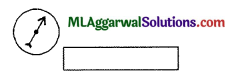(c) What does an electric circuit mean?
(d) Calculate the number of electrons constituting one coulomb of charge.
(e) How much work is done in moving a charge of 2 Colomb from a point at 95 volt to a point at 105 volt?
(a) (i) Long piece of nichrome wire; (ii) Thin piece of nichrome wire.
(b) The completed diagram of the compass and magnet is as follows :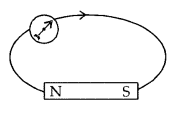(c) A continuous conducting path consisting of wires and other resistances (like electric bulb, etc.) and a switch, between the two terminals of a cell or battery, along which an electric current flows, is called an electric circuit.(d) Charge of an electron is 1.6 x 10-19
If charge is 1.6 x 10-19 C, then no. of electrons = 1
So, if charge is 1 C, the number of electrons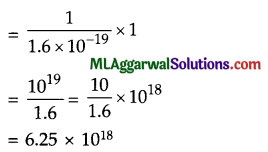Thus, 6.25 x 1018 electrons taken together constitute 1 coulomb of charge

(e)Section – II
(Attempt any four questions from this section)

Question 5.
(a) State three requirements for selecting a unit of a physical quantity.
(b) A ball falls from the top of tower of height 19.6 cm. Find the time of fall and velocity of the ball when it reaches the ground, (g = 8 m/s2)
(c) Name four parts of a vernier callipers and state their functions.
(a) Requirements of standard unit are :
(1) It should be well defined.
(2) It should be easily reproducible at all places.
(3) It should not change with time, and from place to place

(b) Given : u = 0, h =6 m, g = 9.8 ms-2
v2 = u2 + 2gh v
$$v=\sqrt{2 \times 9.8 \times 19.6}$$(c) The four parts of a vernier callipers along with their functions are :
(1) Outside jaws : To measure the length of a rod, diameter of a sphere, external diameter of a hollow cylinder etc.
(2) Inside jaws : To measure the internal diameter of a hollow cylinder or pipe.
(3) Strip : To measure the depth of a beaker or a bottle.
(4) Vernier scale : Helps to measure length correct upto 0.1 mm.Question 6.
(a) Show that : Rate of change of momentum = Mass x Acceleration.
(b) Two balls A and B of masses ‘m’ and ‘2m’ are in motion with velocities 2v and v
Compare : (i) their inertia, (ii) their momentum and (iii) the force needed to stop them in the same time.
(c) A cricket ball of mass 100 g moving with a speed of 40 ms-1 is brought to rest by a player in 0.04 s.
Find : (i) the change in momentum of ball,
(ii) the average force applied by the player.
(a) Let a force ‘F’ be applied on a body of mass ‘m’ for time T due to which its velocity changes from ‘u’ to ‘ν’. then,
Initial momentum of the body = mu
Final momentum of the body = mv
Change in momentum of the body in t second = mv – mu = m (υ – u)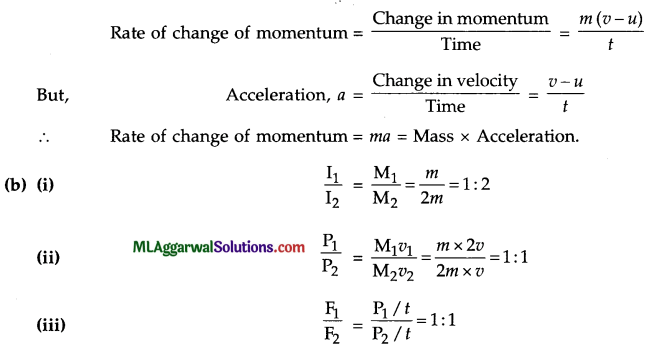(c) Given: m 100g= kg=$$\frac{100}{1000}$$ 0.1kg ,u=40ms-1 v = 0,t = 0.04s.
(i) Initial momentum mu = 0.1 x 40 = 4.0 kg ms-1
Final momentum = mv =1 x 0 = 0
∴ Change in momentum = Final momentum – Initial momentum
= 0 – 4 = – 4kg ms-1
Change in momentum

(ii) Average force, $$\text { Average force, } \mathrm{F}=\frac{\text { Change in momentum }}{\text { Time }}$$
$$=\frac{-4}{0.04}=-100 \mathrm{~N}$$
The negative sign here shows that the force is applied in a direction opposite to the direction of motion of the ball.

Question 7.
(a) A man standing in front of a special mirror finds his image having a very small head, a fat body and the legs of normal size. What are the shapes of the three parts of the mirror ?
(b) An object is placed at a distance of 10 cm from a convex mirror of focal length 15 cm. Find the position and nature of the image.
(c) When a spherical mirror is held towards the sun and its sharp image is formed on a piece of carbon paper for some time, a hole is burnt in the carbon paper.
(d) What is the nature of the spherical mirror ?
(e) Why is a hole burnt in the carbon paper ?
(f) At which point of the spherical mirror is the carbon paper placed ?
(g) What name is given to the distance between the spherical mirror and carbon paper ?
(a) The top part of the special mirror is convex as it produces a small image of the head. The middle part of the mirror is concave as it produces a magnified image of the body. The lower part of the mirror is a plane mirror as it produces the image of the same size.

(b) Given : Object distance, u = – 10 cm
Image distance, ν = ?
Focal length, f = 15 cm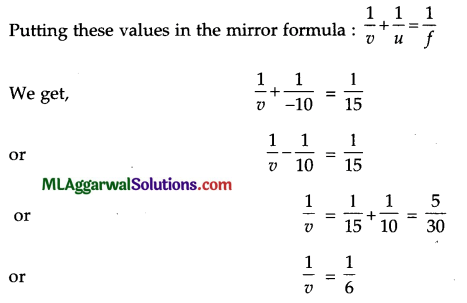So, image distance, v = 6 cm. Thus, the position of image is at a distance of 6 cm from the convex mirror on its right (behind the mirror) since the image is formed behind the convex mirror, therefore, the nature of image is virtual and erect.

(c) (i) Concave mirror,
(ii) A lot of sun’s heat rays are concentrated at the point which bum the hole in carbon paper
(iii) At the focus
(iv) Focal length.Question 8.
(a) State three conditions for the propagation of sound.
(b) State three uses of ultrasonic vibrations.
(c) A student made an electric circuit shown below to measure the current through two lamps.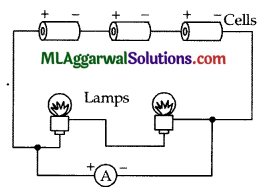(i) Are the lamps in series or parallel ?
(ii) The student has made a mistake in this circuit. What is it ?
(iii) Draw a diagram to show the correct way to connect the circuit. Use the proper circuit symbols in your diagram.
(a) Conditions for the propagation of sound are :
(1) There must be a vibrating body, capable of transferring energy.
(2) There must be a material medium, through which energy can propagate.
(3) There must be a receiver (ear), so as to receive and transmit the energy to the brain.

(b) Ultrasonic vibrations are used in
(1) Dissipating of fog,
(2) Ultrasound welding, and
(3) Ultrasound scanning of the human body.

(c) (i) In series.
(ii) Ammeter is connected in parallel with the lamps. It should be connected in series.
(iii) The corrected diagram is :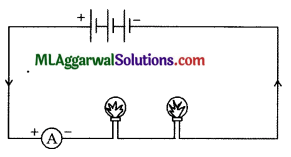Question 9.
(a) State the rules for drawing geometric images in convex mirrors.
(b) Write three equations of uniformly accelerated motion relating the initial velocity (u), final velocity (ν), time (t), acceleration (a) and displacement (s).
(c) Waves A and B as shown in the figure, propagate in the same medium. Frequency of wave A is 5000 Hz. Find the velocity of both waves and frequency of wave B.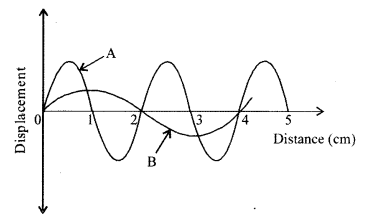Rules for drawing geometric images in convex mirrors :
(1) Any ray of light, travelling parallel to the principal axis of convex mirror, after reflection, appears to pass through the principal focus of the mirror.
(2) Any ray of light travelling along the principal focus of convex mirror, aftrer reflection, travels parallel to the principal axis.
(3) Any ray of light travelling along the centre of curvature of a convex mirror, after reflection, retraces its path.(b) The three equations of motion are :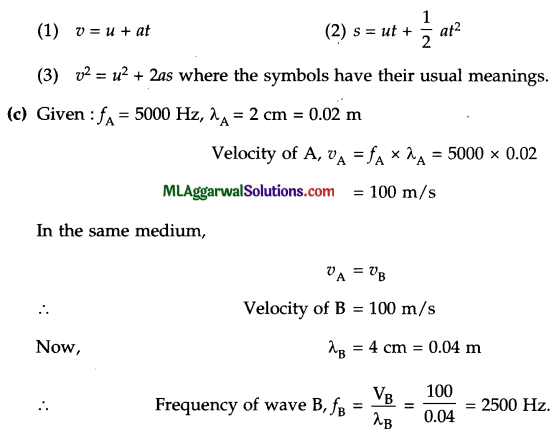Question 10.
(a) You are required to make an electromagnet from a soft iron bar by using a cell, an insulated coil of copper wire and a switch. Draw a circuit diagram to represent the process and label the poles of the electromagnet.

(b) You are given a resistance wire PQ connected with a cell and a key as shown in the given figure. You are required to measure the current in the wire PQ and potential difference across it. Name the instruments that you would use and draw a labelled diagram to show how are they connected. Also, mark the direction of current in each component.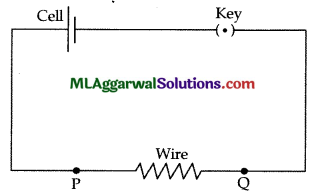(c) A boy measures the length of a piece of pencil by meter rule, vernier callipers and screw gauge to be 7.3 cm, 7.35 cm and 7.353 cm respectively. State (i) the least count of each measuring instrument, (ii) the accuracy in each measurement.
(a) The required circuit diagram is :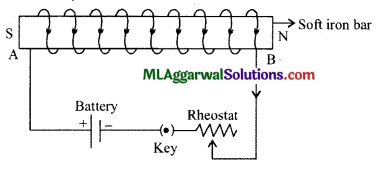(b) An ammeter is used to measure current and a voltmeter is used to measure the potential difference. The ammeter (A) is corrected in series with the resistance wire PQ and cell, while the voltmeter (V) is connected in parallel across the wire PQ.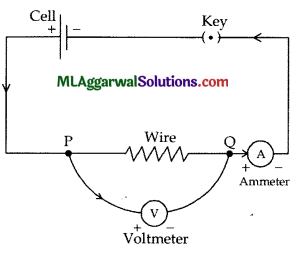(c) (i) Least count of meter rule = 0.1 cm
Least count of vernier callipers = 0.01 cm
Least count of screw gauge = 0.001 cm.

(ii) Accuracy in 7.3 cm = 0.1 cm
Accuracy in 7.35 cm = 0.01 cm
Accuracy in 7.353 cm = 0.001 cm.